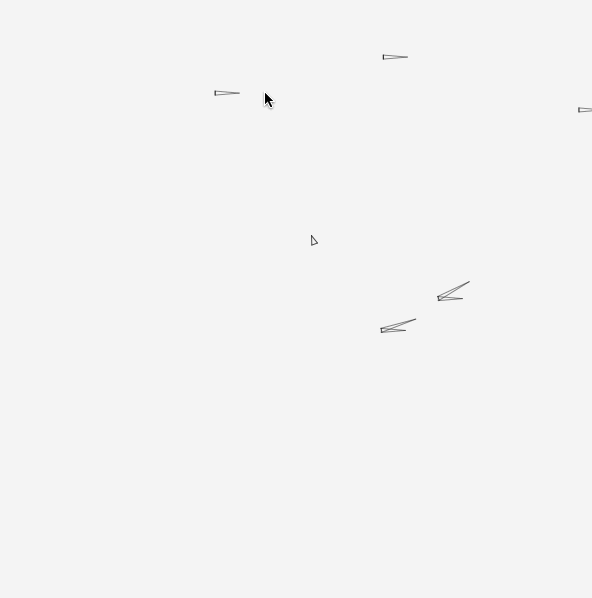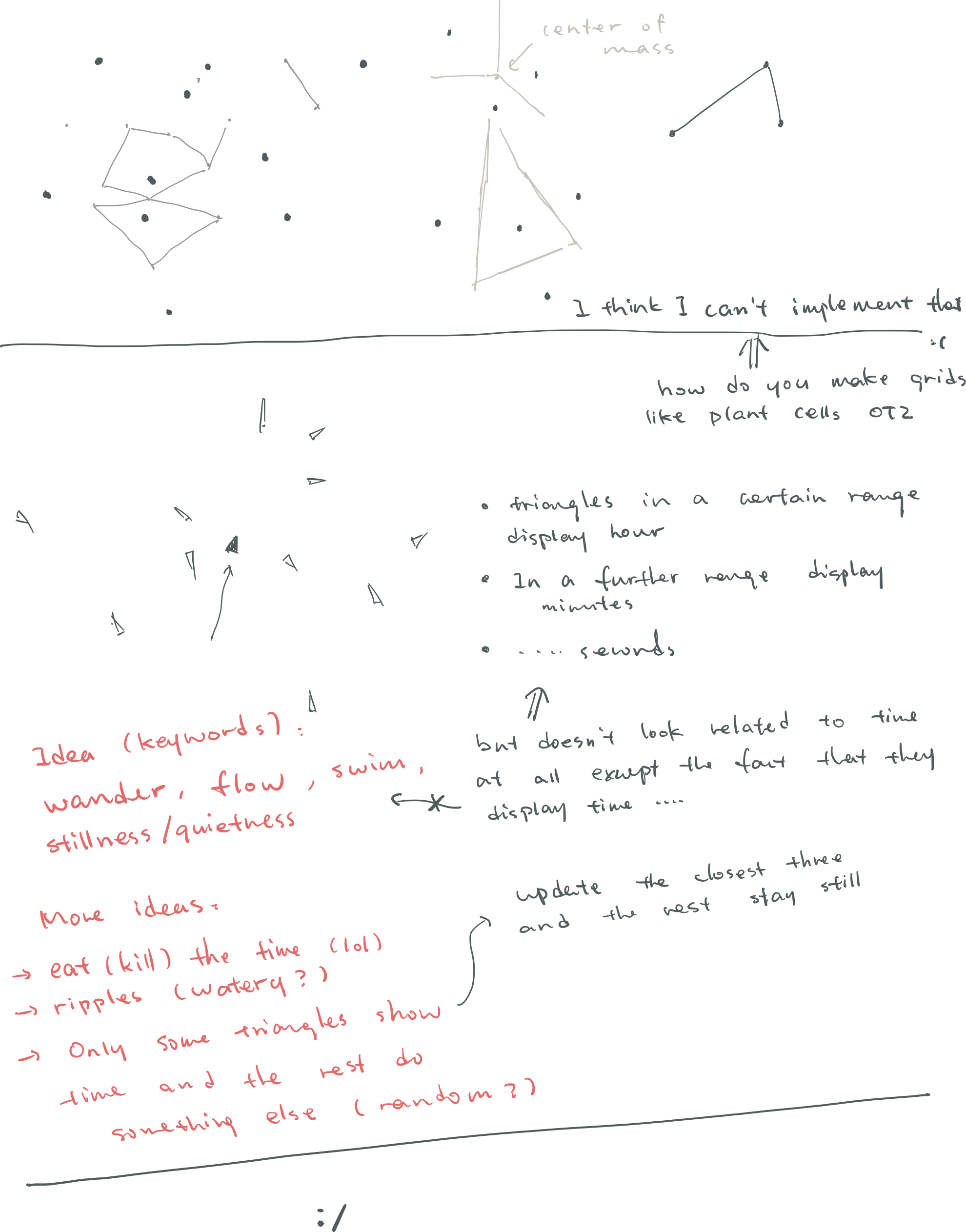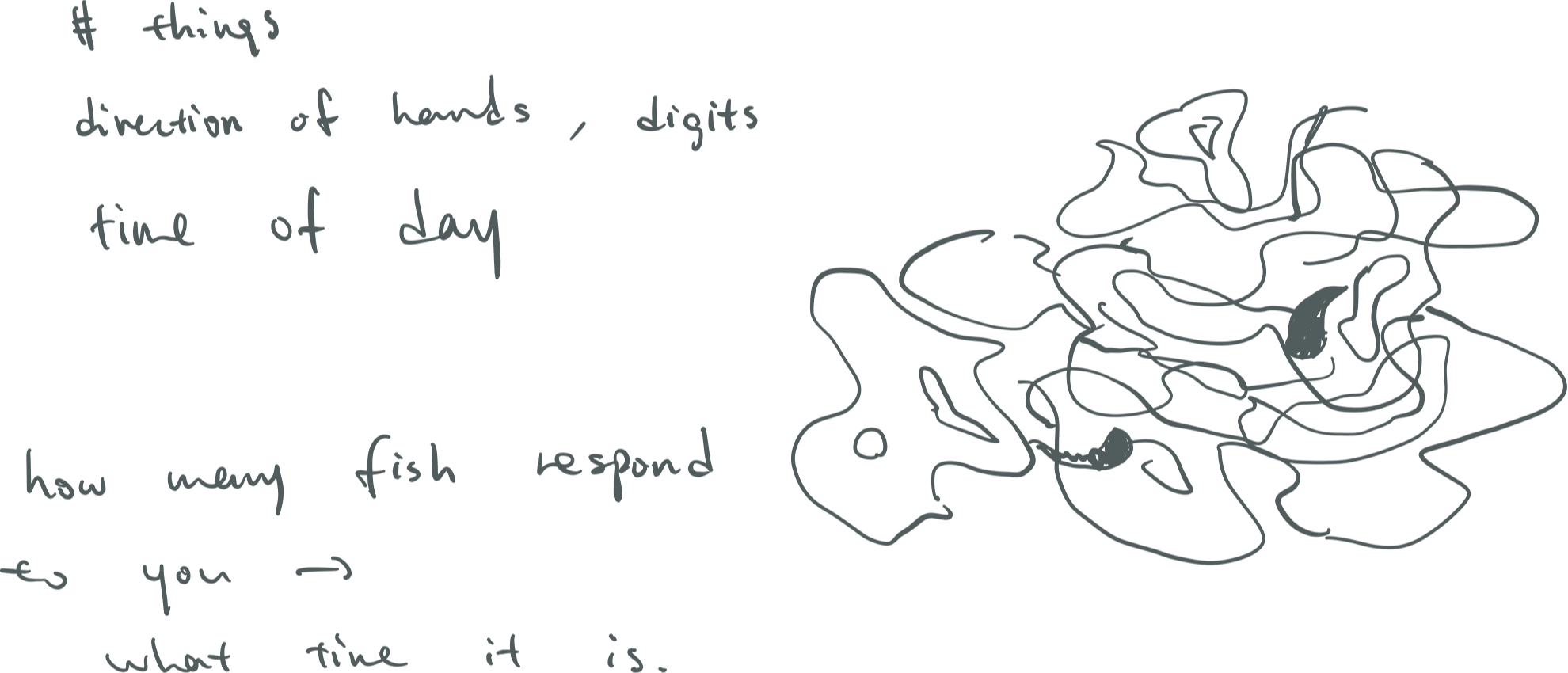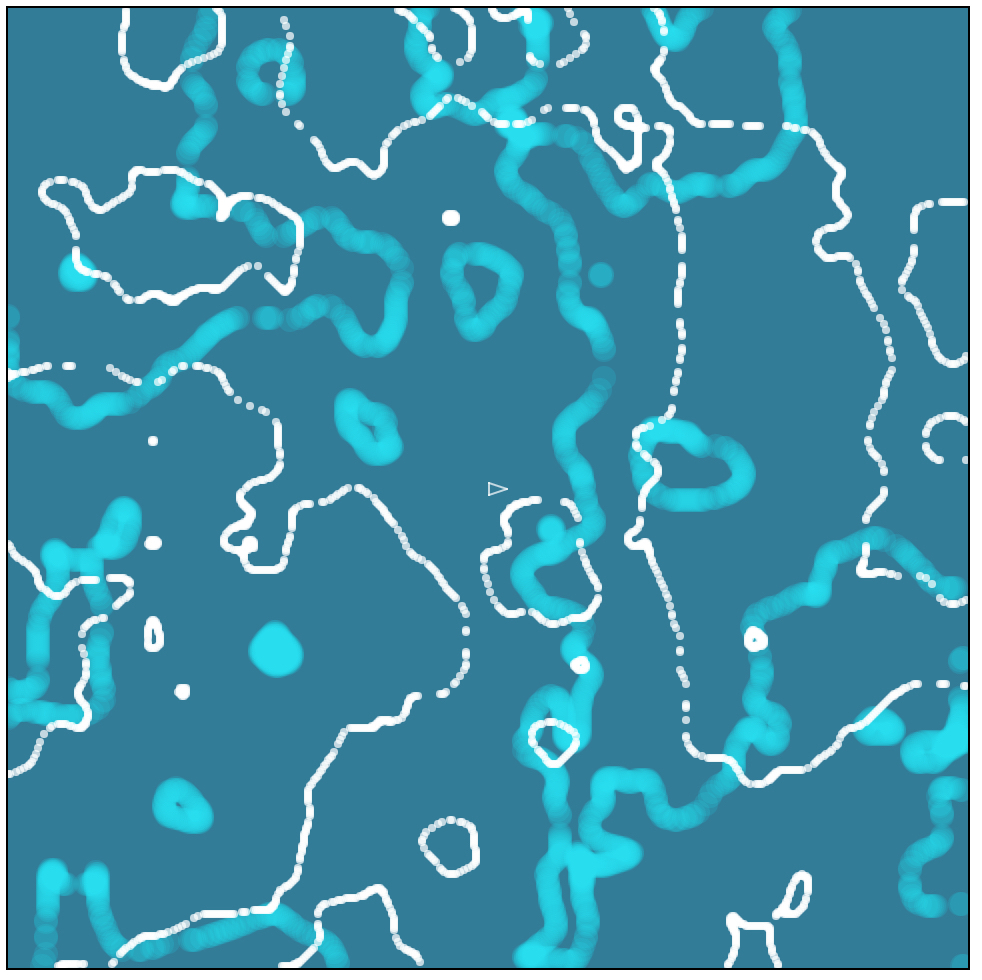# miyehn-Clock

Here it is:

Below is an animated gif:I started out with the idea of drawing clocks on a gridded space, where the space is divided in some natural way like plant cells (see drawing on top). And in each cell there would be a simplistic clock. There would also be a cell – the user – that can navigate around and others would make space for it. Meanwhile the clocks closer to the user would run slower and the ones further from the user would go faster, so that the user would be at the center of “still time”. That’s what I meant when I wrote “standing still”.But there were obstacles. First I tried several ways to decide how to divide space naturally for each clocks, and failed. I didn’t give up the idea of “a space full of clocks”, though, so I implemented the rest anyway, including the movement of user and camera, and the looping, edgeless space. Most of these implementations are still in my final code. I was glad I made all the movement and transitions smooth. Despite the clocks were just randomly distributed in the space.

However the clock hands here and there had no clear indication of time. I experimented around for a while, trying several ways to decide which hands display which part of time – hours, minutes, seconds, or milliseconds. They still don’t make much sense. I thought about changing the color scheme, but then I realized it was probably influenced from Vera Molnar’s Interruptions that made me reluctant.

Thank Golan a lot for pointing out my problems at this moment and giving me some new insights. I really liked his idea of “revealing time”, so that the user would need to do something in order to know the time. Wow this is such a good reason to make anything interactive in general. He also reminded me of how representation of time don’t need to be limited to hour/minute/second standards and use rotating hands or digits to represent them. Some example ideas he offered including a battling spaceship and a crow using pebbles to drink water in a bottle (I was really amazed by how he could come up with ideas so fast).After talking to Golan I made this new sketch of fish in a pond that would swim to the user when the user clicks (throws bread crumbles into the pond), and the number of fish that respond would correspond to the time in a day. I started it but I ran into technical difficulties again – for example, when I tried to simulate water ripples using perlin noise, the program would run really slowly, like at ~10 frame rate.Reflecting back, despite the time limit that made me unable to complete the pond idea, I also felt how difficult it’s going to be to make realistic things with p5 or processing. Golan’s narratives were really nice and poetic, so did the pond idea to some extend. But the canvas in p5 or processing is ultimately a 2D space, and if the imageries cannot be simplified into graphical primitives, it’ll take a lot of resources to render them properly, or would require me to load external images. Even after that, there’s also going to be problems of integration between the realistic images with graphical shapes, as well as the integration between the static and moving parts of the work. That’s a lot to learn and think about….

Eventually I rolled back to my previous idea and left my work within the 2D world of itself, only changing the clock hands again so they now do look more like some representation of time (I hope). Now I understand the potential problems of making realistic narratives through pure coding, so for now I think I’ll just leave the clocks with their own physics rules (although they don’t make total sense in real life). The very least of my original idea “a borderless space of time” was preserved, and although other explorations didn’t make into this piece, I’ve definitely learned new things and gained new perspectives. They will help me in the future works.

```var clocksH; var clocksM; var user; var bleed = 200;   var toCenter; var center; var target; var currentStep;   var H; var M;   function setup() { createCanvas(600,600); noStroke(); center = createVector(width/2,height/2); toCenter = createVector(0,0); currentStep = createVector(); target = createVector(width/2,height/2); clocksH = new Array(); clocksM = new Array(); for(var i=0; i&lt;15; i++) { var x = -bleed+(width+2*bleed)*random(); var y = -bleed+(height+2*bleed)*random(); clocksH.push(new Clock('H', x, y)); clocksM.push(new Clock('M', x, y)); } user = new User(); }   function draw() { H = hour(); M = minute();   background(245); user.update(); currentStep.add(p5.Vector.sub(toCenter,currentStep).mult(0.008));   push(); translate(currentStep.x, currentStep.y); for(var i=0; i&lt;clocksH.length; i++) { clocksH[i].update(); clocksH[i].display(); } for(var i=0; i&lt;clocksM.length; i++) { clocksM[i].update(); if(p5.Vector.sub(user.position, clocksM[i].position).mag()&lt;150){ clocksM[i].mode = 'M'; } else clocksM[i].mode = 'still'; clocksM[i].display(); } user.display(); pop(); }   function clockSorter(C1, C2) { var c1 = p5.Vector.sub(user.position, C1.position).mag(); var c2 = p5.Vector.sub(user.position, C2.position).mag(); return c1-c2; }   class Clock { constructor(mode, x, y) { this.len = 20; this.prevLen = 20; this.curLen = 20;   this.position = createVector(x, y); this.c = color(0, 120); this.mode = mode;   this.targetR = HALF_PI; this.prevCurrentR = HALF_PI; this.currentR = HALF_PI; } update() { this.wrapAround(); this.prevCurrentR = this.currentR; this.prevLen = this.len; if(this.mode=='H') { this.len = 24; this.targetR = H/12*TWO_PI; } else if(this.mode=='M') { this.len = 36; this.targetR = (M)/60*TWO_PI; } else if(this.mode=='S'){ this.len = 16; this.targetR = (S)/60*TWO_PI; } else if(this.mode=='m'){ this.len = 16; this.targetR = (mils)/1000*TWO_PI; } else if(this.mode=='still'){ this.len = 0; this.targetR = H/12*TWO_PI; } var tochange = this.targetR-this.currentR; var tolengthen = this.len-this.curLen; while(tochange&lt;0) tochange+=TWO_PI; this.currentR += tochange*0.05; this.curLen += tolengthen*0.05; }   wrapAround(){ var tmp = p5.Vector.add(this.position, currentStep); if(tmp.x &lt; -bleed) this.position.x += bleed*2+width; if(tmp.x &gt; width+bleed) this.position.x -= bleed*2+width; if(tmp.y &lt; -bleed) this.position.y += bleed*2+height; if(tmp.y &gt; height+bleed) this.position.y -= bleed*2+height; }   display() { this.drawShape(this.position.x,this.position.y, this.currentR); }     drawShape(x, y, r) { push(); translate(x, y); rotate(r-HALF_PI); stroke(this.c); strokeWeight(1); line(0,2, this.curLen,0); line(this.curLen,0, 0,-2); line(0,-2, 0,2); pop(); }   }   class User { constructor() { var x = width/2; var y = height/2; this.position = createVector(x, y); this.v = createVector(); } update() { this.v = p5.Vector.sub( target, this.position ).mult(0.02); this.position.add(this.v); }   display() { this.drawShape( this.position.x, this.position.y, this.v.heading() ) }   drawShape(x, y, r) { push(); translate(x, y); rotate(r); stroke(0,180); strokeWeight(1); line(0,3, 9,0); line(9,0, 0,-3); line(0,-3, 0,3); pop(); }   }   function mod2pi(num) { while(num&gt;TWO_PI){ num-=TWO_PI; } return num; }   function keyTyped() { if(key=='0'){ noLoop(); } else if(key=='1'){ loop(); } }   function mouseClicked() { target = createVector(mouseX, mouseY).sub(currentStep); toCenter = p5.Vector.sub(center, target); }```